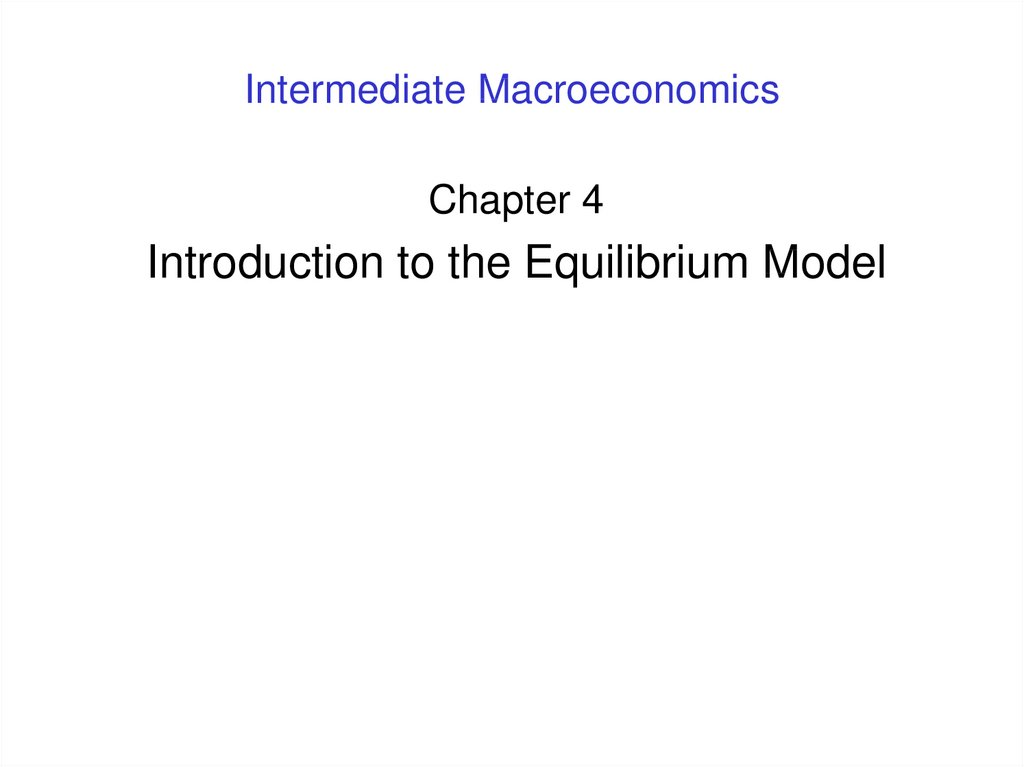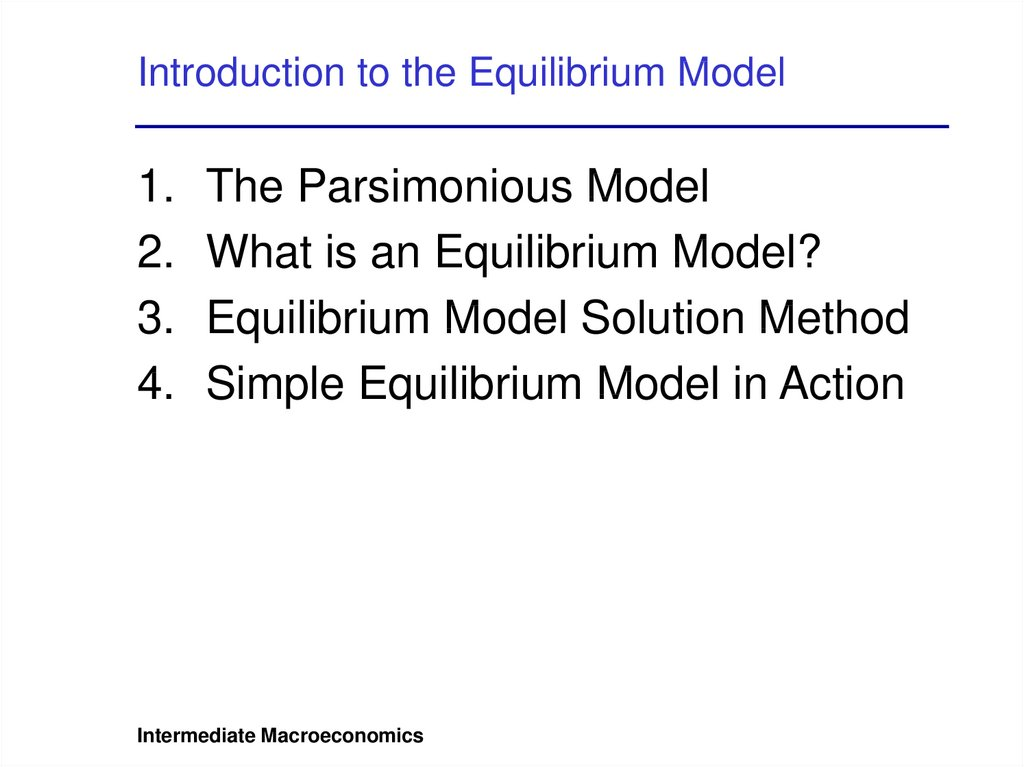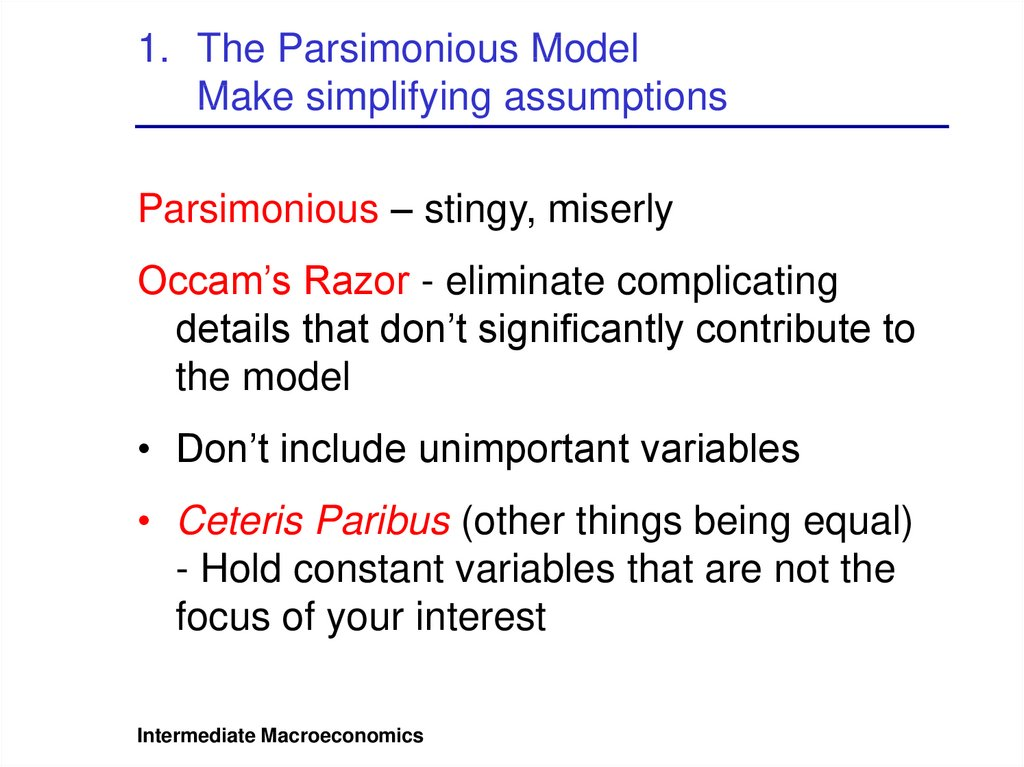# Intermediate macroeconomics. Introduction to the equilibrium model

## 1. Intermediate Macroeconomics

Chapter 4
Introduction to the Equilibrium Model

## 2. Introduction to the Equilibrium Model

1.
2.
3.
4.
The Parsimonious Model
What is an Equilibrium Model?
Equilibrium Model Solution Method
Simple Equilibrium Model in Action
Intermediate Macroeconomics

## 3. The Parsimonious Model Make simplifying assumptions

1. The Parsimonious Model
Make simplifying assumptions
Parsimonious – stingy, miserly
Occam’s Razor - eliminate complicating
details that don’t significantly contribute to
the model
• Don’t include unimportant variables
• Ceteris Paribus (other things being equal)
- Hold constant variables that are not the
Intermediate Macroeconomics

## 4. The Parsimonious Model Simplifying assumptions for our models

1. The Parsimonious Model
Simplifying assumptions for our models
Aggregate output ≡ National income
National income ≡ Personal income
Intermediate Macroeconomics

## 5. What is an Equilibrium Model? Assumed equilibrium condition

2. What is an Equilibrium Model?
Assumed equilibrium condition
• GDP Accounting (Chapter 2):
National Income ≈ Aggregate Supply
• Macroeconomic Models:
Aggregate Supply (AS) = Aggregate Demand (AD)
or
National Income (Y) = Aggregate Demand (AD)
Intermediate Macroeconomics

## 6. What is an Equilibrium Model? Disequilibrium

2. What is an Equilibrium Model?
Disequilibrium
• Disequilibrium: aggregate output (or
national income) is not equal to aggregate
demand
• Undesired Inventory Accumulation: a
symptom of disequilibrium where
aggregate output > aggregate demand
• Undesired Inventory Draw: a symptom
of disequilibrium where
aggregate output < aggregate demand
Intermediate Macroeconomics

## 7. 3. Equilibrium Model Solution Method

1. Substitute the given equations into the
2. Apply the assumed equilibrium condition:
3. Substitute the derived equation for AD
from step 1 into the right-hand side of the
equilibrium condition in step 2.
4. Simplify the equation. This often means
solving for income (Y), since Y should
appear on both the left- and right-hand
sides of the equation in step 3.
Intermediate Macroeconomics

## 8. 4. Simple Equilibrium Model in Action Describing the economy

AD = C + I + G + NX
C = consumption
I = investment
D = government spending
NX = net exports (exports – imports)
YD = C + S
YD = disposable income
S = savings
YD = Y + TR – TA
Y = national income
TR = government transfer payments
TA = government taxes
Intermediate Macroeconomics

## 9. 4. Simple Equilibrium Model in Action Solving the model

1. Substitute given equations into equation for AD:
YD = YD
C + S = Y + TR – TA
C = Y + TR – TA - S
AD = C + I + G + NX
= (Y + TR - TA - S) + I + G + NX
2. Apply equilibrium condition:
3. Substitute solution for AD from Step 1:
Y = Y + TR - TA - S + I + G + NX
4. Simplify equation:
G + TR - TA = S - I - NX
Intermediate Macroeconomics

## 10. 4. Simple Equilibrium Model in Action Implications of the model

In equilibrium:
G + TR - TA = S - I - NX
• Crowding Out
• Ricardian Equivalence
• Twin Deficits
Intermediate Macroeconomics

## 11. 4. Simple Equilibrium Model in Action Crowding Out

In equilibrium: G + TR - TA = S - I - NX
Assume:
– Increase in government deficit (G + TR - TA)
– Savings (S) and net exports (NX) constant
Result:
– Decrease in investment (I)
Intermediate Macroeconomics

## 12. 4. Simple Equilibrium Model in Action Ricardian Equivalence

In equilibrium: G + TR - TA = S - I - NX
Assume:
– Increase in government deficit (G + TR - TA)
– Investment (I) and net exports (NX) constant
Result:
– Increase in savings (S)
Intermediate Macroeconomics

## 13. 4. Simple Equilibrium Model in Action Twin Deficits

In equilibrium: G + TR - TA = S - I - NX
Assume:
– Increase in government deficit (G + TR - TA)
– Savings (S) and investment (I) constant
Result:
– Decrease in net exports (NX)
Intermediate Macroeconomics

## 14. 4. Simple Equilibrium Model in Action Implications of the model

G + TR - TA = S - I - NX
Implications of an increase in the Government
Budget Deficit, G + TR - TA:
Savings
Investment
Net
Exports
Ricardian
Equivalence
Increase
Assume
Constant
Assume
Constant
Crowding Out
Assume
Constant
Decrease
Assume
Constant
Twin Deficits
Assume
Constant
Assume
Constant
Decrease
Intermediate Macroeconomics